This handy Math in Focus Grade 2 Workbook Answer Key Chapter 14 Practice 2 Reading and Writing Time detailed solutions for the textbook questions.

Write the time in words.

Example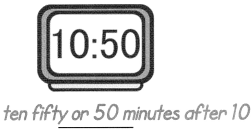Question 1.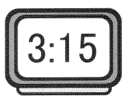three fifteen or 15 minutes after 3.

Question 2.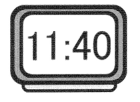eleven forty or 40 minutes after eleven.

Question 3.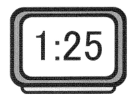one twenty-five or 25 minutes after 1

Question 4.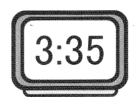three thirty five or 35 minutes after 3

Question 5.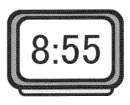eight fifty-five or 55 minutes after 8.

Write the time

Example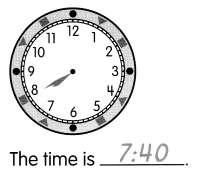Question 6.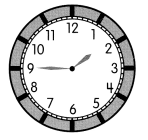The time is _____________.

The time is 1:45

Question 7.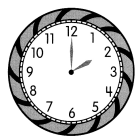The time is _____________.

The time is 2:00

Question 8.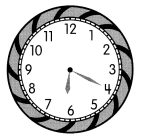The time is _____________.

The time is 6:20

Question 9.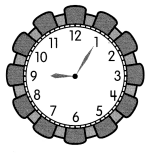The time is _____________.

The time is 9:05

Question 10.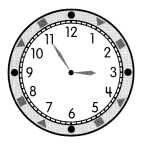The time is _____________.

The time is 2:55

Draw the minute hand to show the time.

Example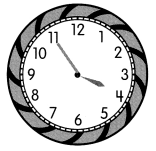The time is 3:55.

Question 11.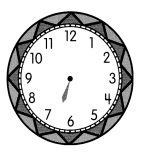The time is 6:30.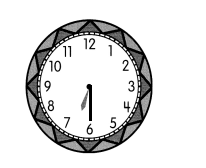Question 12.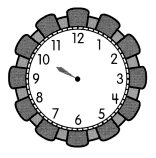The time is 10:15.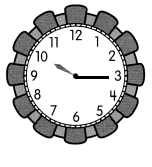Question 13.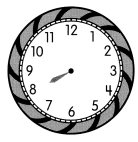The time is 8:00.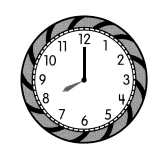Question 14.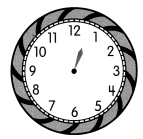The time is 12:40.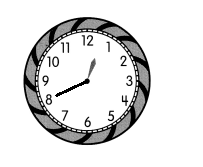Question 15.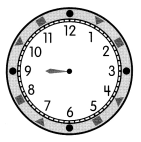The time is 9:05.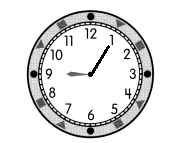Draw the hour hand to show the time.

Example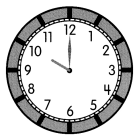The time is 10:00.

Question 16.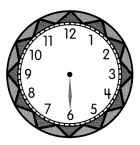The time is 11:30.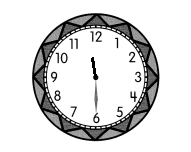Question 17.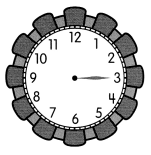The time is 7:15.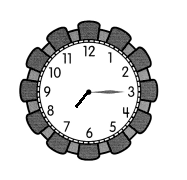Question 18.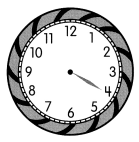The time is 4:20.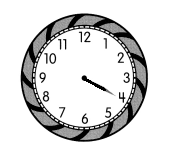Question 19.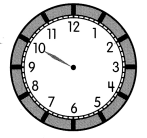The time is 2:50.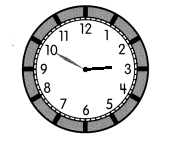Question 20.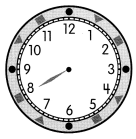The time is 3:40.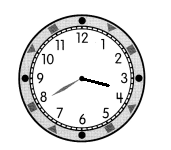Draw the hands to show the time.

Example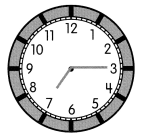The time is 7:15.

Question 21.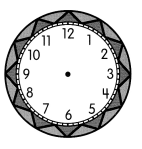The time is 4:30.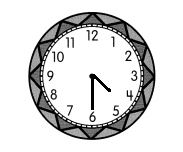Question 22.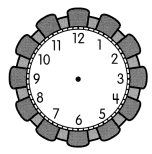The time is 1:20.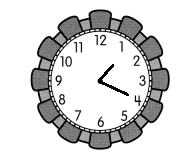Question 23.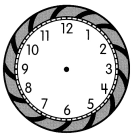The time is 9:25.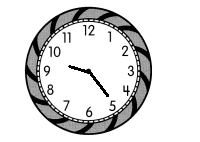Question 24.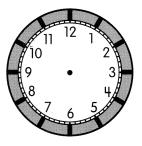The time is 7:00.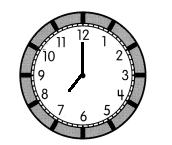Question 25.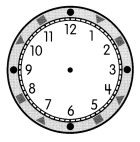The time is 9:50.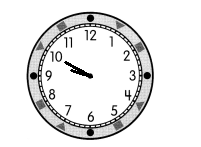Draw the hands to show the time. Then write the time in words.

Example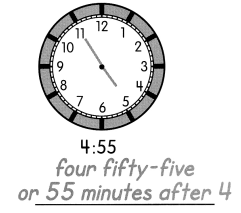Question 26.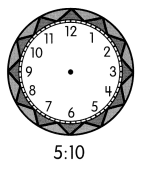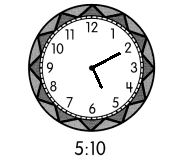five ten or 10 minutes after 5

Question 27.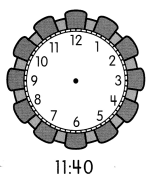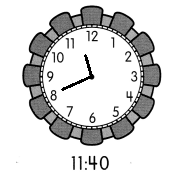eleven forty or 40 minutes after 11

Question 28.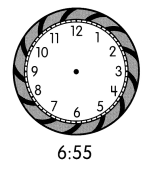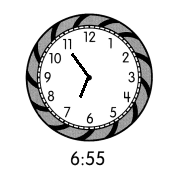six fifty five or 55 minutes after 6

Question 29.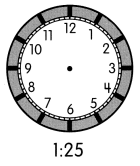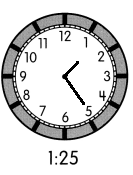one twenty-five or 25 minutes after 1

Question 30.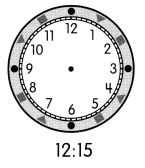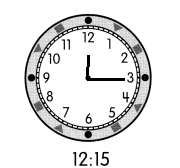twelve-fifteen or 15 minutes after 12